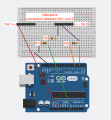# Combining capacitive sensing and ohmMeter with Arduino

#### TillFly

Joined Oct 26, 2016
69
Hey,
i´m looking for a circuit combining capacitive sensing and an ohmMeter to create an interface.
my prototype has two cupper contact foils. when you connect both of them one can measure the resistance of whatever conductive you put in between.
now i want to add a capacitive sensor for each of the two foils.

the attached picture shows my current circuit.

the base values with no touch are :
• A0 of ohmMeter = 0
• D2 (CapSense) = 135

if i touch the capsense with my hand:
• D2 (capSense value) jumps to ca. 2700

if i additionally connect the two foils with two fingers
• D2 (capSense value) jumps to around 20000, why that?
• A0 of ohmmeter jumps to 135
when i put my whole hand on both foils the serial monitor stucks and jumps from values like 300000 to -2#include <CapacitiveSensor.h> CapacitiveSensor cs_4_2 = CapacitiveSensor(4,2); // 10 megohm resistor between pins 4 & 2, pin 2 is sensor pin, add wire, foil int analogPin = 0; int raw = 0; void setup() { cs_4_2.set_CS_AutocaL_Millis(0xFFFFFFFF); // turn off autocalibrate on channel 1 - just as an example Serial.begin(9600); } void loop() { // RESISTANCE SENSING raw = analogRead(analogPin); Serial.print("raw: "); Serial.println( raw ); // CAPACITIVE SENSING long start = millis(); long total1 = cs_4_2.capacitiveSensor(30); Serial.print(millis() - start); // check on performance in milliseconds //Serial.print("\t"); // tab character for debug window spacing Serial.print("CAP_SENSE: "); Serial.println(total1); // print sensor output 1 delay(10); // arbitrary delay to limit data to serial port }